# The pool

The pool contains 220 m3 of water. The pool can be emptied either:
a) 10 hours of pipe B and 8 hours of pipe A, or
b) 10 hours of pipe A and 7 hours of pipe B.

How many cubic meters of water will flow in 1 hour from pipe A and how many from pipe B?

A =  15
B =  10

### Step-by-step explanation:

10•B + 8•A = 220
10•A + 7•B = 220

8A+10B = 220
10A+7B = 220

A = 15
B = 10

Our linear equations calculator calculates it.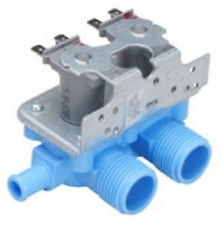Did you find an error or inaccuracy? Feel free to write us. Thank you!Tips to related online calculators
Looking for calculator of harmonic mean?
Looking for a statistical calculator?
Do you have a system of equations and looking for calculator system of linear equations?
Tip: Our volume units converter will help you with the conversion of volume units.

## Related math problems and questions:

• The poolThe pool has a volume of 40 m3, and the water temperature is 20 °C. How much water at 100 °C should we pour into the pool to increase the water temperature by 5 °C?
• Two water containers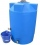In the first container, there are 200 m3 of water and in the second 40 m3. The first container will flow down at a rate of 10 m3 water per hour. At the same time flows to the second rate of 5 m3 per hour. After how many hours there will be three times les
• Three pumps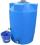The water tank is emptied with the first pump in 6 hours, with the second pump in 2 hours. In order to be emptied in 1 hour, all three pumps had to be started at once. How long will take tank be emptied by only the third pump?
• Water pool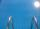Pool with volume 990hl completely filled, if water flows by one tap 8 hours and by second tap 6 hours. First tap give 10hl more than second per hour. How many hl flows in each of them in an hour?
• Water reservoir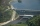One drain hole emptied the water from the tank for 20 hours, with the second drain for 30 hours. How long will the water drain from this tank if we open two drains simultaneously?
• PoolIf water flows into the pool by two inlets, fill the whole for 19 hours. The first inlet filled pool 5 hour longer than the second. How long pool take to fill with two inlets separately?
• Tributaries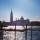The first tributary fill pool with water in 15 hours. The second tributary fill pool in 10 hours. For how many hours the pool is filled with both tributaries?
• Pond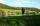A pond with two open floodgates will emptied in 20 days. A larger floodgate will emptied whole pond in 30 days. For how long would he be emptied with a smaller floodgate.
• Tanker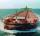An oil tanker can be emptied by the first pump in 4.9 hours. The second pump can empty a tanker in 8 hours. If the first pump started at 4:00 and the second 1.4 hour later, at what time will the tanker be empty?
• Water current speed.Two cities along the river are 100 km apart. The powerboat downstream runs for 4 hours, upstream for 10 hours. Determines the river's current speed.
• Two pipes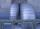One pipe fill one-fifth volume 20 minutes before by second one. The two pipes together will fill the tank in two hours. How long is will fill tank each pipe separately?
• The poolThe cube-shaped pool has 140 cubic meters of water. Determine the dimensions of the bottom if the depth of the water is 200 cm and one dimension of the bottom is 3 m greater than the other. What are the dimensions of the pool bottom?
• Two valves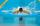Water fills the pool with two valves for 11 days. After 7 days, the first valve was stopped, and the second valve fill pool for 7 days. How many days took to fill the pool of each of the valves individually?
• PipesThe water pipe has a cross-section 1087 cm2. An hour has passed 960 m3 of water. How much water flows through the pipe with cross-section 300 cm2 per 9 hours if water flows the same speed?
• Aircraft angines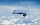The two engines of the aircraft are enough to supply the fuel for five hours of operation. However, one of the engines has a malfunction and thus consumes one-third more fuel. How long can the plane be in the air before it runs out of fuel? After an hour
• 3 pupils3 pupils cleaned 10 meters of the stream in 1 hour. How many meters of the stream will clean 18 students in 4 hours?
• Mixture of coffee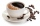They have to prepare a mixture of coffee in packing centres so that 1 kg costs 240 CZK. There are two types of coffee in stock at the price of 220 CZK per 1 kg and 300 CZK per 1 kg. How many kilograms of each species need to be mixed to prepare 50 kg of t